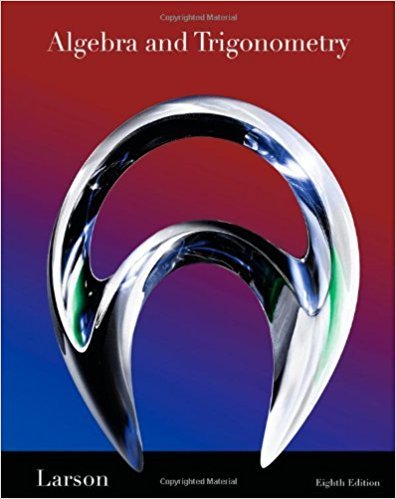×
×

# Solutions for Chapter 7.3: Solving Trigonometric Equations## Full solutions for Algebra and Trigonometry | 8th Edition

ISBN: 9781439048474Solutions for Chapter 7.3: Solving Trigonometric Equations

Solutions for Chapter 7.3
4 5 0 370 Reviews
31
4
##### ISBN: 9781439048474

This expansive textbook survival guide covers the following chapters and their solutions. Chapter 7.3: Solving Trigonometric Equations includes 107 full step-by-step solutions. Since 107 problems in chapter 7.3: Solving Trigonometric Equations have been answered, more than 49498 students have viewed full step-by-step solutions from this chapter. Algebra and Trigonometry was written by and is associated to the ISBN: 9781439048474. This textbook survival guide was created for the textbook: Algebra and Trigonometry, edition: 8.

Key Math Terms and definitions covered in this textbook
• Basis for V.

Independent vectors VI, ... , v d whose linear combinations give each vector in V as v = CIVI + ... + CdVd. V has many bases, each basis gives unique c's. A vector space has many bases!

• Cofactor Cij.

Remove row i and column j; multiply the determinant by (-I)i + j •

• Column space C (A) =

space of all combinations of the columns of A.

• Commuting matrices AB = BA.

If diagonalizable, they share n eigenvectors.

• Complete solution x = x p + Xn to Ax = b.

(Particular x p) + (x n in nullspace).

• Hypercube matrix pl.

Row n + 1 counts corners, edges, faces, ... of a cube in Rn.

• Identity matrix I (or In).

Diagonal entries = 1, off-diagonal entries = 0.

• Jordan form 1 = M- 1 AM.

If A has s independent eigenvectors, its "generalized" eigenvector matrix M gives 1 = diag(lt, ... , 1s). The block his Akh +Nk where Nk has 1 's on diagonall. Each block has one eigenvalue Ak and one eigenvector.

• Linearly dependent VI, ... , Vn.

A combination other than all Ci = 0 gives L Ci Vi = O.

• Norm

IIA II. The ".e 2 norm" of A is the maximum ratio II Ax II/l1x II = O"max· Then II Ax II < IIAllllxll and IIABII < IIAIIIIBII and IIA + BII < IIAII + IIBII. Frobenius norm IIAII} = L La~. The.e 1 and.e oo norms are largest column and row sums of laij I.

• Orthogonal matrix Q.

Square matrix with orthonormal columns, so QT = Q-l. Preserves length and angles, IIQxll = IIxll and (QX)T(Qy) = xTy. AlllAI = 1, with orthogonal eigenvectors. Examples: Rotation, reflection, permutation.

• Pivot columns of A.

Columns that contain pivots after row reduction. These are not combinations of earlier columns. The pivot columns are a basis for the column space.

• Pivot.

The diagonal entry (first nonzero) at the time when a row is used in elimination.

• Positive definite matrix A.

Symmetric matrix with positive eigenvalues and positive pivots. Definition: x T Ax > 0 unless x = O. Then A = LDLT with diag(D» O.

• Rotation matrix

R = [~ CS ] rotates the plane by () and R- 1 = RT rotates back by -(). Eigenvalues are eiO and e-iO , eigenvectors are (1, ±i). c, s = cos (), sin ().

• Semidefinite matrix A.

(Positive) semidefinite: all x T Ax > 0, all A > 0; A = any RT R.

• Toeplitz matrix.

Constant down each diagonal = time-invariant (shift-invariant) filter.

• Trace of A

= sum of diagonal entries = sum of eigenvalues of A. Tr AB = Tr BA.

• Transpose matrix AT.

Entries AL = Ajj. AT is n by In, AT A is square, symmetric, positive semidefinite. The transposes of AB and A-I are BT AT and (AT)-I.

• Tridiagonal matrix T: tij = 0 if Ii - j I > 1.

T- 1 has rank 1 above and below diagonal.

×